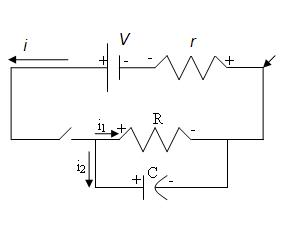# Using Kirchoff's Laws to solve a circuit

## Homework Statement

Find i, i1, i2 in terms of V, r, R, c, and t on the following circuit:## Homework Equations

I suppose I would use Kirchoff's law for this...

## The Attempt at a Solution

i = i1 + i2
-ir + V – i1R = 0
-ir + V – Vc = 0

Vc = V – ir = q/c
i1R = -ir + V

Where do I go from here?

Following the path with just the resistor and the capacitor can I reduce the current to the equation: i2 = (V/r)e^(-t/rC)

After the transient current that charges the capacitor has gone, you have 2 resistors in series so

V = i ( r + R)

You can get the transient by calculating the voltage across R.

This is what I'm currently thinking...

i= [V/(r+R)](1-e^-(t/rC))+(V/r)e^-(t/rC)
i1= [V/(r+R)](1-e^-(t/rC))
i2= (V/r)e^-(t/rC)